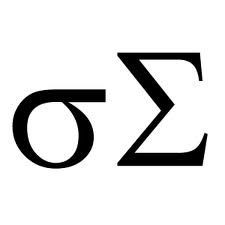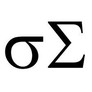# How to Calculate Standard Deviation

## Step-by-step tutorial for computing the standard deviation of a set of numbers with an example.

The standard deviation is a statistic, a number that is calculated from another set of numbers.  Other statistics include the mean and median, which tell you where the "center" is in a set of data. The standard deviation tells you how spread out the data is, how much it deviates from the center.  People who crunch numbers for a living use statistical calculators and software to compute the standard deviation, but if you know the formula you can also compute it with a simple hand calculator.

### The Formula

The formula for the standard deviation of a set of numerical data is sqrt(∑(m-xi)2/n), where m is the mean of the set, the xi are the elements of the set, and n is the number of elements in the set.  To be more accurate, this is the formula for population standard deviation.  You use this formula when your data represents the entire sample space.

If your set of data is a merely a sample from a larger set, the formula for standard deviation is slightly different: sqrt(∑(m-xi)2/(n-1)). This is called, conveniently, the sample standard deviation. Notice that instead of dividing by n, you divide by n-1.This has the effect of slightly increasing the standard deviation. The larger the value of n, the less of a difference this makes.

### Sample Exercise in Computing the Standard Deviation

Alice and Bob are thinking about selling their home.  To come up with a reasonable selling price, they take a sample of other homes in their city that are for sale and are of a similar size and age.  Here are the numbers they come up with:

• \$100,000
• \$140,000
• \$150,000
• \$150,000
• \$175,000
• \$190,000
• \$230,000

The mean selling price of the 7 homes is \$162,143.  Since this is a sample of a larger set, they should use the second formula, sqrt(∑(162143-xi)2/6).  The final answer is \$41,217.

### Another Example

An anthropologist surveys all of the adult residents in a small village to find out how many different languages people can speak.  Out of the 1000 adults, 681 speak only one language, 205 speak two languages, 109 speak three languages, 4 speak four languages, and 1 person can speak six languages.

The mean number of languages spoken by a person is [681*1 + 205*2 + 109*3 + 4*4 + 1*6]/1000 = 1440/1000 = 1.44.

The population standard deviation is sqrt(∑(1.44-xi)2/1000), or

sqrt[(681*(1.44-1)2 + 205*(1.44-2)2 + 109*(1.44-3)2 + 4*(1.44-4)2 + 1*(1.44-6)2)/1000]

= sqrt[(131.84 + 64.29 + 255.53 + 26.21 + 20.79)/1000]

= sqrt[498.66/1000]

= 0.7062.

4

Only logged-in users are allowed to comment. Login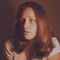EKlein on 01/19/2012

Good explanation of the difference between population s.d. and sample s.d.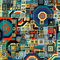Yes, they can do that, or they can consider +/- 1 standard deviation from the mean to be an "average" range. Realistically, though, their sample size is pretty small and they'd be better off contacting a real estate agent!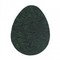TerriRexson on 01/13/2012

So how do Alice and Bob use the standard deviation to come up with a selling price for their house? Do they add the standard deviation to the mean and then go a bit under that to be at the higher end of normal?

#### You might also like

Buttons from the Rag Bag! Button Jar Math Activities

Save the buttons from discarded clothing and recycle them as fun, hands-on ma...

Kumon Math Review

A mother's review of the Kumon Math program including suggestions for kumon a...

Disclosure: This page generates income for authors based on affiliate relationships with our partners, including Amazon, Google and others.